Download Presentation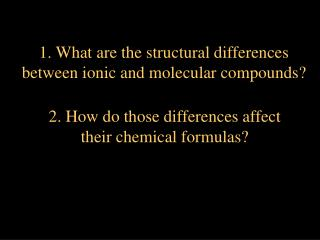1. What are the structural differences between ionic and molecular compounds?

# 1. What are the structural differences between ionic and molecular compounds? - PowerPoint PPT Presentation

1. What are the structural differences between ionic and molecular compounds?. 2. How do those differences affect their chemical formulas?. The chemical formula for a covalent compound is called a molecular formula ;. The chemical formula for an ionic compound is called a formula unit.I am the owner, or an agent authorized to act on behalf of the owner, of the copyrighted work described.
Download Presentation## 1. What are the structural differences between ionic and molecular compounds?

An Image/Link below is provided (as is) to download presentation

Download Policy: Content on the Website is provided to you AS IS for your information and personal use and may not be sold / licensed / shared on other websites without getting consent from its author.While downloading, if for some reason you are not able to download a presentation, the publisher may have deleted the file from their server.

- - - - - - - - - - - - - - - - - - - - - - - - - - E N D - - - - - - - - - - - - - - - - - - - - - - - - - -
Presentation Transcript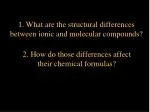1. What are the structural differences

between ionic and molecular compounds?

2. How do those differences affect

their chemical formulas?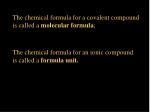The chemical formula for a covalent compound

is called a molecular formula;

The chemical formula for an ionic compound

is called a formula unit.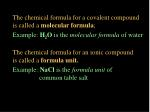The chemical formula for a covalent compound

is called a molecular formula;

Example: H2O is the molecular formula of water

The chemical formula for an ionic compound

is called a formula unit.

Example: NaCl is the formula unit of

common table salt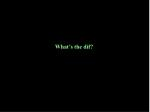A nice

VISUAL look

at the difference

will help out: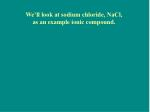We’ll look at sodium chloride, NaCl,

as an example ionic compound.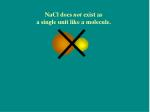NaCl does not exist as

a single unit like a molecule.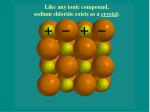Like any ionic compound,

sodium chloride exists as a crystal:The formula unit for sodium chloride is NaCl.

However, if we look inside the crystal,

the Na+ and Cl- ions are each bonded

to six oppositely charged neighbors:Let’s shrink

the ions so

we can take

a peek insideLet’s shrink

the ions so

we can take

a peek insideWe’ll take a

look at this

Cl- ion.How many

Na+ ions is

the Cl- close

enough to

bond with?We’ll start

again

from theWe’ll start

again

from theAnd,

how many

Cl- ions is

each Na+ ion

bonded to?Because each Na+ is bonded to more than one Cl- ion,

and each Cl- is bonded to more than one Na+ ion, thenall the ions in the whole crystal are

part of one single bonded unit,

and the crystal below would be Na32Cl32.This is impractical, given the enormous numbers

of ions in a crystal large enough to see with our eyes.

So, only the ratio of ions is given (its empirical formula),

which is called a formula unit.

NaCl is the formula unit for

the sodium chloride crystal.

NaCl is the smallest whole number

ratio of sodium and chloride ions

in the crystal.This is impractical, given the enormous numbers

of ions in a crystal large enough to see with our eyes.

So, only the ratio of ions is given (its empirical formula),

which is called a formula unit.

NaCl is the formula unit for

the sodium chloride crystal.

NaCl is the smallest whole number

ratio of sodium and chloride ions

in the crystal.CaF2

2+

1-water is a molecule.

‘space filling’ model ‘ball and stick’ modelwater is covalently bonded hydrogen and oxygen.

‘space filling’ model ‘ball and stick’ modelIn large amounts of H2O, any attraction between

H2O molecules is due to weak forces, and so the

molecules behave as individual units, not one large

bonded unit as in an ionic crystal.This difference in structure between molecules and

ionic compounds is reflected in their symbols:H2O

NaCl

This difference in structure between molecules and

ionic compounds is reflected in their symbols:

The molecule is represented by a molecular formula,

showing all the atoms present in the unit.

The ionic compound is represented

by the ratio of its ions, which is called a formula unit.

versusIN SUMMARY, in a molecule, the atoms given by

the formula are all covalently bonded. In a collection

of those molecules, the molecules are attracted to

each other by weak forces, but no covalent bonds

occur between molecules--the molecules exist as

individual units of covalently bonded atoms.

BUT, in an ionic compound, where the bond is the

electrostatic attraction between oppositely charged

ions, each ion is bonded to each of its oppositely

charged neighbors!

So, for any ionic compound, all the ions in the whole

crystal can be thought of as part of one single bonded unit.

So the formula unit shows the lowest whole number ratio

of the ions, rather than representing ALL the ions in the crystal.